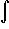#Interactive Real Analysis

Next | Previous | Glossary | Map

## 7.2. Integration Techniques

### Example 7.2.4(d): Applying the Substitution Rule

Findtan(x) dx andcot(x) dx
This will be a relief because it is easy: tan(x) = sin(x)/cos(x) so that the top function is the derivative of the bottom function (except for a minus sign).

Therefore we can apply a previous example (or make the substitution u = cos(x)) to determine the answer right away:tan(x) dx = -ln(cos(x))
To findcot(x) dx is left as an (easy) exercise.
Next | Previous | Glossary | Map